# Discrete

This toolbox contians ten discrete components: Accumulator, Integrator, Unit Delay, Delay, Transport Delay, Rate Limiter, Differentiator, FIR Filter, Discrete Transfer Function, Discrete State Space, and the Hysteresis Function.

The Discrete toolbox provides ten discrete components, such as Accumulator, Delay, Integrator, etc. Their descriptions and supported features are given in Table 1.

Table 1. Discrete components in HIL Signal Processing Toolbox
Component Description Features Help file
supported input types supported output types vector support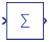Accumulator

Accumulates an input signal. real, int, uint real, int, uint yes Accumulator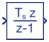Integrator

Integrates an input signal using the Backward Euler method. real, int, uint real yes Integrator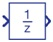Unit Delay

Delays the input signal by one sample period. real, int, uint real, int, uint, inherit (user selectable) yes Unit Delay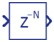Delay

Delays the input signal by N sample periods. N can be specified through a property (fixed delay) or by the input signal (variable delay). real, int, uint real, int, uint, inherit (user selectable) yes Delay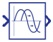Transport Delay

Applies a defined delay to the input signal. real, int, uint real, int, uint, inherit (user selectable) yes Transport delay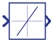Rate Limiter

Limits the rising and falling rate of the change. real, int, uint real, int, uint yes Rate Limiter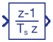Differentiator

Provides a derivative of the input signal. real, int, uint real yes Differentiator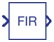FIR Filter

Implements a direct form FIR filter. real, int, uint real, int, uint, inherit (user selectable) yes FIR Filter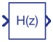Discrete Transfer Function

Implements a discrete transfer function where the numerator and the denominator can be specified in either the Z or S domain. real, int, uint real, int, uint, inherit (user selectable) yes Discrete Transfer Function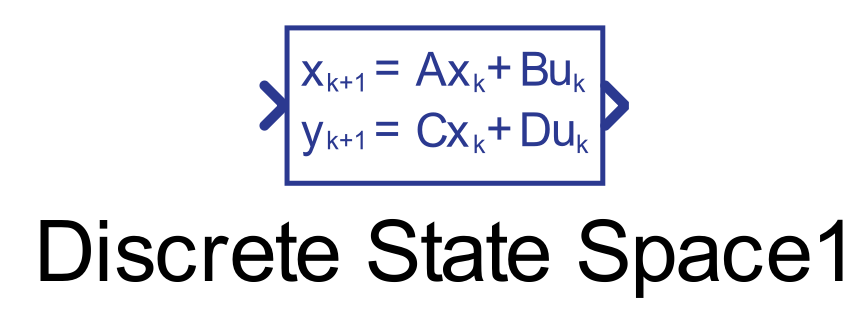Discrete State Space

Implements a discrete state space where coefficient matrices A,B,C and D can be specified in either the Z or S domain. real, int, uint real, int, uint, inherit (user selectable) yes Discrete State Space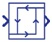Hysteresis Function

Models a hysteresis function. real, int, uint real, int, uint yes Hysteresis Function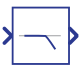Low-Pass Filter

Models a low-pass filter. real, int, uint real yes Low-Pass Filter